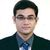Announcements
You can succeed best and quickest by helping others to succeed. Join the conversation.
cancel
Showing results for
Did you mean:Anonymous
Not applicable

## Bug in set analysis?

Hi All,

I have this data set:

 Scenario Version Product Amount Actual 1 A 10 Actual 2 A 9 AG 1 A 12 AG 2 A 11 AG 3 A 13 FC 1 A 9 FC 2 A 10

In a straight table, product as dimension and expression as Sum({\$<Product=,Scenario={'Actual'},Version={"\$(=Max(Version))"}>} Amount), it's giving no result. If I select in Scenario Actual of FC, it's giving correct result that is 9. But if i select Scenario as AG, it's not giving any result again. Something to do with possible max version when selection is made. When nothing is selected, it's taking max(version)=3, and there is no actual for 3.

Thanks

SAK

1 Solution

Accepted SolutionsMVP

The dollar sign expansion of \$(=Max(Version)) will be performed before the actual expression will be evaluated, hence only once per chart and not considering the dimension. It's only considering user selection state.

If you need the max version per dimensional value, you can for example use FirstsortedValue:

FirstSortedValue( {\$<Product=,Scenario={'Actual'} >} Amount, -Version)

Or if you want to find the max version of Scenario Actual, you can use a set expression within the dollar sign expansion;

Sum({\$<Product=,Scenario={'Actual'},Version={"\$(=Max({<Scenario = {'Actual'}>} Version))"}>} Amount)

2 RepliesHi,

It is not the issue with Set analysis. It is with what you want.

If you can explain what you want probably that will help us to suggest you solution.

Regards,

Kaushik Solanki

Regards,
KaushikMVP

The dollar sign expansion of \$(=Max(Version)) will be performed before the actual expression will be evaluated, hence only once per chart and not considering the dimension. It's only considering user selection state.

If you need the max version per dimensional value, you can for example use FirstsortedValue:

FirstSortedValue( {\$<Product=,Scenario={'Actual'} >} Amount, -Version)

Or if you want to find the max version of Scenario Actual, you can use a set expression within the dollar sign expansion;

Sum({\$<Product=,Scenario={'Actual'},Version={"\$(=Max({<Scenario = {'Actual'}>} Version))"}>} Amount)Community Browser